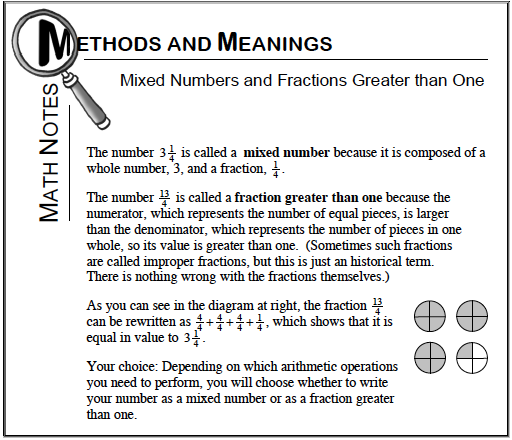### Home > CC1 > Chapter 5 > Lesson 5.1.2 > Problem5-17

5-17.

Convert each mixed number to a fraction greater than one, or each fraction greater than one to a mixed number. Homework Help ✎

1. $5 \frac { 3 } { 11 }$

1. $\frac { 49 } { 4 }$

1. $3 \frac { 1 } { 20 }$

1. $\frac { 603 } { 100 }$

See the Math Notes box below to help you convert each number.Remember to draw a diagram if that is helpful for you.

$12\frac{1}{4}$

For parts (c) and (d), refer to the Math Notes box.